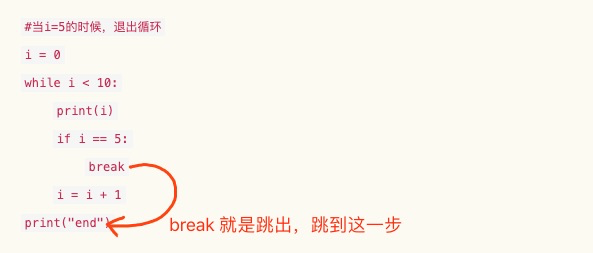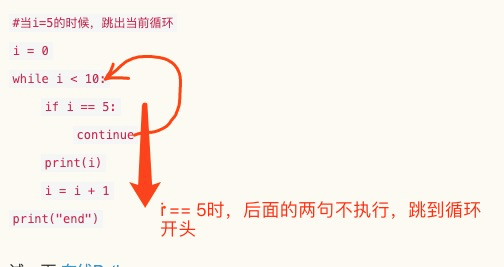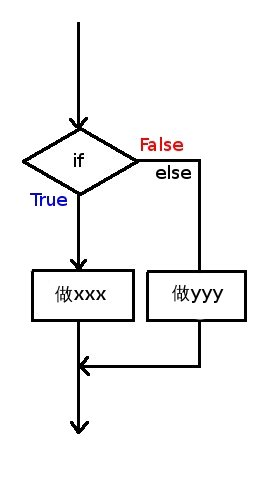《金哥百变Python零基础教程7》~实战I

# 本章知识点 :> `编程实战`# 孰能生巧

• 前面3章我们学习了 `函数 列表 字典` 相关知识。

• 编程就是一个熟练的过程。
• 针对这些知识，我们在看几个小问题

• 1/ 打印n以内的奇数，n是一个整数，要求用函数实现。
• 按照惯例，先定义名字 `n以内奇数发现法`
• 输入:n *输出：打印所有数

`#还记得如何定义函数吗 （第5章知识)`
`def findji(n):`
`#先定义一个数初始值为1`
`i = 1`
`#循环`
`#重复判断i是不是为奇数，当然i要不断加1`
`while i < n:`
`if i % 2 == 1:#判断奇数的方法`
`print(i)`
`i = i +1 #别忘记`
`#没有return`

• 2/ 写一个函数，要求随机生成一个数a （0-100）再生成一个数b(0-100), 再随机的做一次四则运算(+ - * /)，打印算式和结果
• 按照惯例，先取函数名：`“随机四则运算器"`
• 输入： 无
• 输出： 打印算式和结果

• 思路：随机我们可以用random.randint函数

• 思路：运算随机怎么做呢？一般方法是这样，我们可以把 `+ - * /` 存入一个列表
• `list = ['+', '-', '*', '/']`
• 此时我们用randint函数去随机生成下标（0，1，2，3），再用下标取出运算符

• `import random #注意import`
`def rand_jisuan():`
`a = random.randint(0,100)`
`b = random.randint(0,100)`
`list = ['+', '-', '*', '/']`
`i = random.randint(0,3)`
`op = list[i]`
`#现在注意：不能直接用 a op b 的语句哦~（op是个字符串)`
`if op == '+': #要用if语句`
`print(str(a) + op + str(b) + "=" + str(a+b))`
`if op == '-': #要用if语句`
`print(str(a) + op + str(b) + "=" + str(a-b))`
`if op == '*': #要用if语句`
`print(str(a) + op + str(b) + "=" + str(a*b))`
`if op == '/' and b != 0: #要用if语句,注意b不能等于0`
`print(str(a) + op + str(b) + "=" + str(a/b))`

试一下 在线Python
• 3/ 假设我们有 地球文 和 外星文的 对照表，如下：(要求实现一个翻译函数从 地球文可以翻译到外星文)

#### "我" -》"查" ， "爱" =》 "踢" ，"你" =》 "岗"

• 取名：`外星文翻译器`
• 输入: "我爱你" （中文)
• 输出：”查踢岗“ (外星文)
• 思路：我们可以用字典把对应关系存储起来（"我" -》"查" ， "爱" =》 "踢" ，"你" =》 "岗" ）
• 思路：我们可以循环一个字符串(`for in `语句，后面字符串部分会再讲)
• `for s in "我爱你":`     `print(s)`
• 上述的代码会遍历字符串并打印每一个字符

`def fanyi(zhongwen):`
`d = {'我':'查', '爱':‘踢’, '你':'岗'}`
`waixin = '' #建立一个字符串存储外星文`
`for s in zhongwen:`
`w = d[s] #查出字典`
`waixin = waixin + w #把翻译出来的字加到waixin后面`
`return waixin`

# 几个补充语法

• 上面的几个问题都可以自己独立写出来了吗？
• 如果还不能也没关系，跟着写出之后，可以回头去尝试默写一遍。

• 下面讲几个跟 `循环` `if` `函数` 相关的补充语法，很多时候使用这些语法会方便很多！

• 你只要有一个印象，之后遇到类似问题可以回来查一查。

• 1 / `break``continue`

• 我们可以在 `循环` 里控制循环的跳出 (`while` `for`)
• 这句话不好理解，可以用图示的方法来做一个说明

### break

`#break直接跳出循环`

`#当i=5的时候，退出循环`
`i = 0`
`while i < 10:`
`print(i)`
`if i == 5:`
`break`
`i = i + 1`
`print("end")`

• 图示• 思考一下：这个程序执行会打印什么？（打印 0， 1，2，3，4 之后就跳出了）
试一下 在线Python

### continue

`#continue跳出当次循环继续下一次`
`#continue跟break很像`

`#当i=5的时候，跳出当前循环`
`i = 0`
`while i < 10:`
`if i == 5:`
`continue`
`print(i)`
`i = i + 1`
`print("end")`

• 图示一下• 不知道为什么叫`continue` 这个名字，总之，`continue` 不是完全跳出循环，而是跳到循环开头(也就是不执行continue后面的语句)

• 思考一下：上面的代码。当`i == 5``continue` 会发生什么？（会发生死循环，因为i一直等于5，没机会+1)
试一下 在线Python

• 修改一下：

• `#当i=5的时候，跳出当前循环`
`i = 0`
`while i < 10:`
`i = i + 1`
`if i == 5:`
`continue`
`print(i)`
`print("end")`
• 这次不会`死循环`了, 输出 1,2,3,4,6,7,8,9,10 (没有5，因为当i==5时跳过print语句）
试一下 在线Python

• 2 / `if else 分支语法`

• 之前说的 `if`，是：`“如果”`条件满足，就做`xxx`

• `a = 10`
`if a < 100:`
`print("a < 100")`
• `if else` 表示的意思是 `“如果”`条件满足，就做`xxx``否则` 就做 `yyy`

• `a = 10`
`if a < 100:`
`print("a < 100")`
`else:`
`print("a >= 100")`

试一下 在线Python

• 注意哦，`if``else` 两个代码段只会执行1个

• 思考：这段代码输出什么？
• 现在改一下代码：

`a = 10`
`if a < 100:`
`print("a < 100")`
`print("a >= 100")`
• 这次你再思考一下会输出什么？再改一下a=100，由输出什么?
试一下 在线Python

• 来个图示会更好理解# 数据结构

• 我们平常总说，程序由 `数据结构``算法` 组成
• 其实写程序也很像是写文章，`数据结构` 就是文章里的 `名词` ,`算法` 就是`动词`
• 我们学过的基本数据有 `整数` `字符串` `浮点数` `布尔值` `None` `列表` `字典`
• 很有意思的是，现实中的数据不管是什么结构，都可以用这些类型组合表示。
• 我们并不缺表示方法，只是缺少从现实问题设计出合适的 `结构` 的能力。

• 比如：如何表示1号线和2号线合起来的地铁线路？( 更多线路先不考虑，太复杂了)

• 注意：这个结构要方便我们计算最优的地铁问路算法.• 思考一下：先把各个站点简化表示为`a` `b` `...`
• 可以用字典存储吗？
• `d = {'a':'b', 'b':'c'} #这也是之前的表示方法`
• 如果用一个d 来表示 1，2号两个地铁可以吗？（注意：换乘站问题)
• 现在我们注意到，`换乘站` 问题是最关键的。
• 既然不能同时用一个d 表示两条线，那我们就创建2个字典
• `d1 = {'a':'b', 'b':'c'} d2 = {'a1':'b1', 'b1':'c1'}`
• 思考一下: 当问路的起始站 和 终点站不在1条线（比如起始站在1号线，终点在2号线）？
• 这个时候，我们肯定要用上换乘站。所有需要一个结构存储换乘站信息，用什么好呢？
• 可以用列表，`list = ['复兴门', '建国门']`

试一下 在线Python

# 算法

• 问路算法`d1 = {'a':'b', 'b':'c'} #1号线`
`d2 = {'a1':'b1', 'b1':'c1'} #2号线`
`huan = ['复兴门', '建国门']`

• 现在，我们考虑一下问路问题？
• 其中最要紧的是 换乘问题？对吧！
• 如果 起点 和 终点 都在 1条线上，那就是之前的问题
• 如果 起点 和终点 分别在1，2号上呢？
• 那一定要换乘了，从起点 a 到终点b的最短路径是多少？
• 最短路径 是不是 可以先计算a 到换乘站的最短路径，再计算换乘站到b的最短路径？
• a->x (换乘站)最短路径为 s1，x->b 最短路为s2 ，假设 s1+ s2 不是a->b的 最短路径
• 在这个假设之下，一定有有一个s3 比s1小，或s4比s2小，不符合实际，因此s1 + s2 就是最短路径。
• 最后，还得考虑一个特殊情况，假设起点和终点在换乘站上，那就需要分别在1，2号线上计算最短路径。
试一下 在线Python

• 可以定义一个函数 `地铁线路最短路径` （解决1条线的最短路径查询)

• 输入：d start end
• 输出：方向，zou_num

`def ditie_cha(d, start,end): #这里的d是地铁路径`
`#...`

• 再定义一个函数 `1-2号线最短路径`
• 输入： start end
• 输出： print(走法)

`def ditie_line12(start, end):`
`#...`

• 我们知道 `d1` `d2` 只能表示1个方向，还需定义 `d10` `d20` 表示反方向
• 当然最好是有一个函数能转化 `d1` `d2` -> `d10` `d20`
`def ni_d(d): #把d反过来`

## `#...`

• 我们只实现最关键的部分就可以了

# 综合运用

`def ditie_cha(d, start,end): #这里的d是地铁路径`
`num = 0#刚开始为0`
`if (start in d) == False or (end in d) == False: #如果不在这条线上`
`return (None, -1) #-1表示走不到`
`total = len(d) #计算最多走的站数`
`next = start`
`while num < total:`
`next = d[next]`
`num = num + 1`
`if next == end:#如果找到直接返回`
`return (d[‘fangxiang'], num)`
`return (None, -1) #找一遍都没有，返回-1`

`def ni_d(d): #把d反过来`
`#d={'a':'b','b':'c'}`
`d1 = {}`
`for s,n in d.items():`
`d1[n] = s`
`return d1`

`#比较最短路径函数`
`def min(list):`
``

`d1 = {'fangxiang':'右','四惠东':'四惠','四惠':'大望路','大望路':'国贸','国贸':'永安里','永安里':'建国门',`
`'建国门':'东单','东单':'王府井','王府井':'天安门东','天安门东':'天安门西','天安门西':'西单','西单':'复兴路',`
`'复兴路':'南礼士路','南礼士路':'木樨地'}`
`d10 = ni_d(d1)`
`d10['fangxiang'] = '左'`
`d2 = {'fangxiang':'右','前门':'崇文门','崇文门’:'北京站'，'北京站':'建国门','建国门’:'朝阳门'，'朝阳门':'东四十条','东四十条’:'东直门'，'东直门':'雍和宫','雍和宫’:'安定门'，'安定门':'鼓楼大街','鼓楼大街’:'积水潭'，'积水潭':'西直门','西直门’:'车公庄', '车公庄':'阜成门','阜成门':'复兴门','复兴门':'长椿街','长椿街':'和平门','和平门':'前门'}`
`d20 = ni_d(d2)`
`d20['方向'] = '左'`
`huan = ['复兴门', '建国门']`

`def ditie_line12(start, end):`
`global fangxiang,huan,d1,d10,d2,d20`
`#如果正好在换乘站上`
`if huan.index(start) ! = -1 and huan.index(end) != -1:`
`#四个路线尝试`
`f1,n1 = ditie_cha(d1, start, end)`
`f2,n2 = ditie_cha(d10, start, end)`
`f3,n3 = ditie_cha(d2, start, end)`
`f4,n4 = ditie_cha(d20, start, end)`
`f,n = min([[f1,n1],[f2,n2],[f3,n3],[f4,n4]])`

`elif start in d1 and end in d1:`
`f1,n1 = ditie_cha(d1, start, end)`
`f2,n2 = ditie_cha(d10, start, end)`
`f,n = min([[f1,n1],[f2,n2])`

`elif start in d2 and end in d2:`
`f1,n1 = ditie_cha(d2, start, end)`
`f2,n2 = ditie_cha(d20, start, end)`
`f,n = min([[f1,n1],[f2,n2])`

`else: #最后一种情况就是分别在2条线`
`...`

Sorry but the SQLBolt exercises require a more recent browser to run.